# Subtraction + multiplication - math problems

#### Number of problems found: 108

• PLUS MINUS product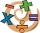A. Deduct 15.728 from the sum of 23.234 and 6.23 B. Add 15.756 to the difference of 26.845 and 4.342 C. Find the product of 0.86 x 0.05 D. Multiply 5.234 by 4.45 E What is the product of 23.97 multiply by 6.36?
• Alica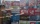Alica went to the market and bought 2 sacks of 50-kilograms of rice that costs P2,200.50 per sack. She wants to sell it in her grocery store at P48.50 per kilo. How much is her profit.
• Paulownia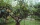Seed germination is 80%. Of the sprouted plants, 8% subsequently die. How many plants does a gardener grow from 1,500 seeds?
• What is 13What is the number sentence? * 1 point 1/2 + 3/4 + 1/4 = A 1/2 - 3/4 - 1/4 = B 1/2 x 3/4 x 1/4 = C 1/2 ÷ 3/4 ÷ 1/4 = D
• One large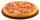One large pizza is cut into 12 pieces. The pizza has sausage, mushrooms, and cheese on it. Michelle ate 1/6 of the pizza, Natalie ate 1/4 of the pizza, Bridgette ate 1/8 of the pizza, and Brenda ate 1/3 of the pizza. How much of the whole pizza is left, a
• Enrollees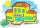The enrolment for this school year in Villahermosa integrated school is 276. If 1/3 are grade ten and are 1/4 grade seven, how many are grade eight and grade nine enrollees?
• Mother 8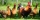Mother bought 2 kg of beef at P240 per kilo and 2 kg of chicken legs at P140 per kilo. She gave the meat vendor a P1000 bill. How much change did she get?
• Mother 7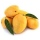Mother bought 18 fruits. 1/3 were pineapple and the rest  were mangoes . how many were mangoes
• Product and sumWhat is the product of two fourths  and the sum of three halves and four?About 6/9 of the sixth- grade pupils will be going to the parents' seminar. If 1/6 of the participants are girls, what part of the portion of sixth graders are boys?
• Nice two numbersWhat two numbers we give a sum of 30 difference of 24 products of 81 and a quotient of 9?
• Nathan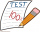Nathan is playing a trivia game. Each correct answer is worth 2 ½ points, and each incorrect answer is worth -¼ points. If he gets 35 questions correct and 32 questions incorrect, how many points does he have? A. 87.5 B. 79.5 C. 75 D. 8
• 30 eggs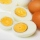There are 30 eggs in a tray. If 1/2 of the tray used 1/5 of it cooked,1/3 kept the refrigerator, how many eggs were left?
• Translate 2Translate the given phrases to mathematical phrases. Thrice the sum of three fifths and two thirds less one half is what number?
• A land 2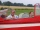A land owner owns two parcels of land measuring 4 2/5 hectares and 6 1/5 hectares. He sells 3/4 of the total land. How many hectares of the land are left?
• Simplify expression with mixedWhen (7¹/3 + 2¾)÷(2 + 2¼ × 1¹/3) is simplified the result is?
• Evaluate 17Evaluate 2x+6y when x=- 4/5 and y=1/3. Write your answer as a fraction or mixed number in simplest form.
• A burger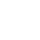The water starts with 41 1/4 cups of water how much water is left after ten 3 5/8 Scoops of water are removed?
• Evaluate fractionsThe difference  of 5 1/2 and 2/3 is added to the product of 5/6 and 1/2
• Fraction expressionWhich expression is equivalent to : minus 9 minus left parenthesis minus 4 start fraction 1 divided by 3 end fraction right parenthesis

Do you have an exciting math question or word problem that you can't solve? Ask a question or post a math problem, and we can try to solve it.

We will send a solution to your e-mail address. Solved examples are also published here. Please enter the e-mail correctly and check whether you don't have a full mailbox.

Subtraction Problems. Multiplication Problems.